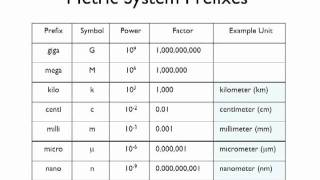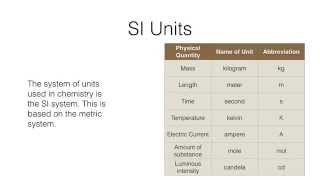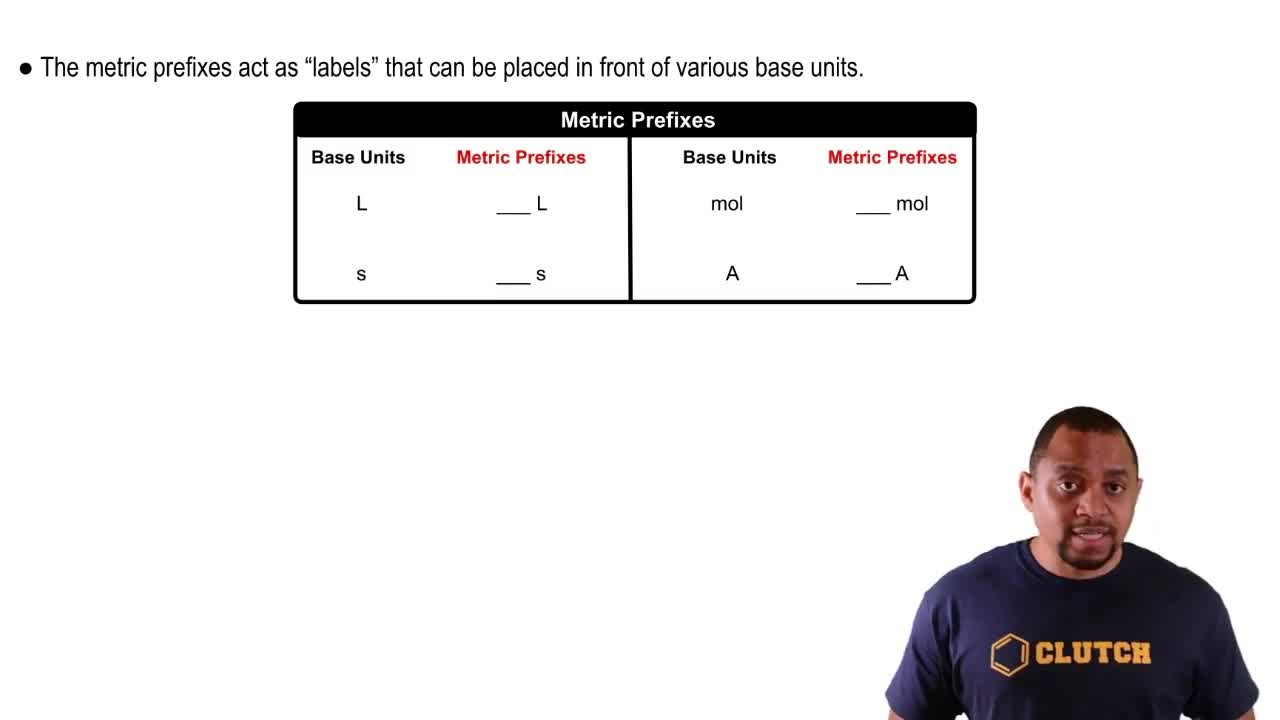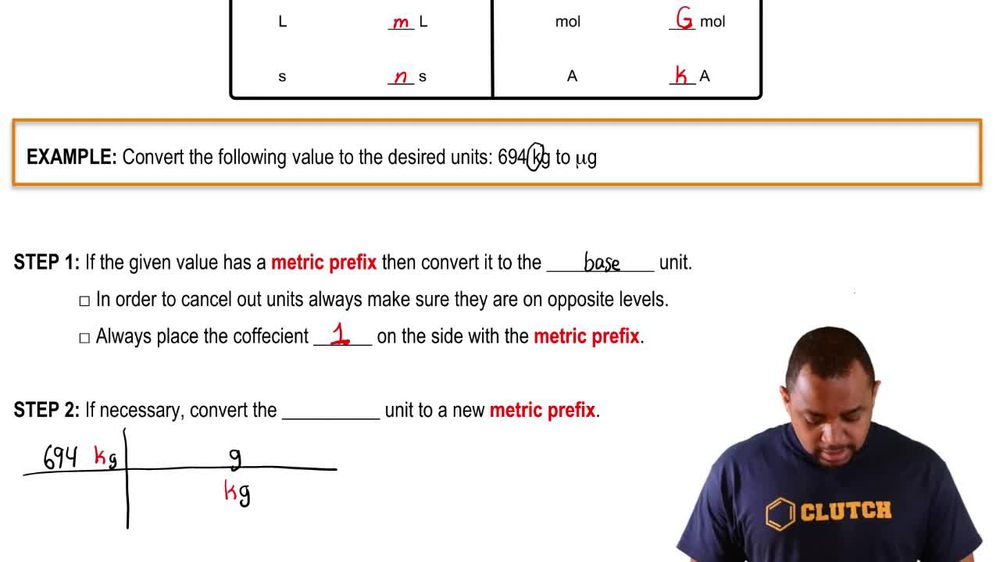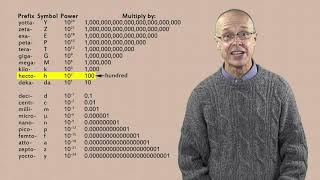Start typing, then use the up and down arrows to select an option from the list.
1. 1. Intro to General Chemistry2. Metric Prefixes
Problem

# Aerogels are transparent, low-density materials that are nearly 99.8% empty space and excellent insulators against hot and cold. The density of a silica-based aerogel is 3.0 mg/cm3. What is the density in units of g/m3? (LO 1.17) (a) 3.0 * 10-3 g/m3 (b) 3.0 * 101 g/m3 (c) 3.0 g/m3 (d) 3.0 * 103 g/m3

Relevant Solution1m
Play a video:
Hello everyone today we have the falling problem. A carbon nanotube is a low density material made of carbon tubes with diameters usually in the nanometer scale. As a strong material with a high tensile strength and elastic modulates the highest density carbon nanotubes fabricated have a density of 1. g per centimeters cubed. Convert this density to kilograms per meters cubed. So first we want to write are given are 1.6 g per centimeter cubed. And we know that we're going to have to convert these grams to kilograms. And in doing that we can use the conversion factor that one kg is equal to 10 to the third grams. Next we have to convert this centimeters cubed, two m cubed. And to do that we use the conversion factor that one centimeter is equal to 10 To the -2 m. And of course because it is in units of Cube, we're gonna get that exponent of three on the outside. And in doing this we're going to see our units cancel out. And we will be left with 1.6 Times 10 to the 3rd kilograms per meters. Cute. And with that we have our answer. I hope this helped until next time